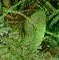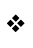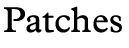Reading digits incorrectly Submitted by: Irene Callaci     Appeared in: Library 6/7 or before     Fixed in: Library 6/10 Problem If the player enters a digit instead of the text version of a number (2 instead of TWO, for example), an incorrect error message is returned ("You can't see any such thing"). The change below allows the player to enter TAKE 2 COINS or TAKE TWO COINS and both versions are recognized. Solution In Parserm.h find the Descriptors() function. The following line: ` n=NumberWord(o);` should be changed to: ` n=TryNumber(wn-1);` in the following block of code: ``` if (allow_plurals && allow_multiple) { n=NumberWord(o); if (n==1) { indef_mode=1; flag=1; } if (n>1) { indef_guess_p=1; indef_mode=1; flag=1; indef_wanted=n; indef_nspec_at=wn-1; indef_type = indef_type | PLURAL_BIT; }```